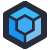Let's say you wanted to express the difference between two numbers as a percentage of the first number. And then let's say that you have no idea how to properly handle a situation where that first number is zero.

In that situation, you might end up with C code much like what Gaetan found.

``````double delta(double number1,double number2)
{
double delta=1e19;
if ( fabs(number1) > 0 )
{
delta =  (number1 - number2)/number1 ;
} else {
delta = number1 - number2 ;
}
delta = fabs(delta);
return delta ;
}
``````

This code is used to "validate" a temporary file by computing a percentage difference between two values. It's part of a "too intricate to be abandoned" pile of C/C++ software written around the turn of the century and gradually expanded over the past two decades.

For such a simple method, I find myself puzzling over nearly every line.

Why is `delta` defaulted to a small number, especially when it's definitely getting set so that value is discarded anyway? Why `fabs(number1) > 0`, and not, I don't know, `number1 != 0`?

But then the real puzzle is in the logic. If `number1` isn't a zero, we can divide by it. But if it is zero we're just going to return the absolute value of `number2`. Which seems like a mistake.

In the specific calling case, Gaetan identifies some more problems:

• the two arguments are physical values. So the result either has the original physical dimension, or no dimension
• The context of the calls clearly expects a number with no physical dimension, so we are bound to encounter problems (or we would have had there been good test cases).
If the file is not valid according to this twisted logic, the caller leaves a whole structure uninitialized[Advertisement] ProGet’s got you covered with security and access controls on your NuGet feeds. Learn more.